# NCERT Solutions for Class 9 Science chapter 11 - Work and Energy - eSaralHey, are you a class 9 student and looking for ways to download NCERT Solutions for Class 9 Science Chapter 11 "Work and Energy"? If yes. Then read this post till the end.

In this article, we have listed NCERT solutions for class 9 Science Physics Chapter 11 in PDF that is prepared by Kota’s top IITian’s Faculties by keeping Simplicity in mind.

If you want to learn and understand class 9 Science chapter 11 "Work and Energy" in an easy way then you can use these solutions PDF.

NCERT Solutions helps students to Practice important concepts of subjects easily. Class 9 Science solutions provide detailed explanations of all the NCERT exercise questions that students can use to clear their doubts instantly.

If you want to score high in your class 9 Science Exam then it is very important for you to have a good knowledge of all the important topics, so to learn and practice those topics you can use eSaral NCERT Solutions.

So, without wasting more time Let’s start.

### Download The PDF of NCERT Solutions for Class 9 Science Chapter 11 "Work and Energy"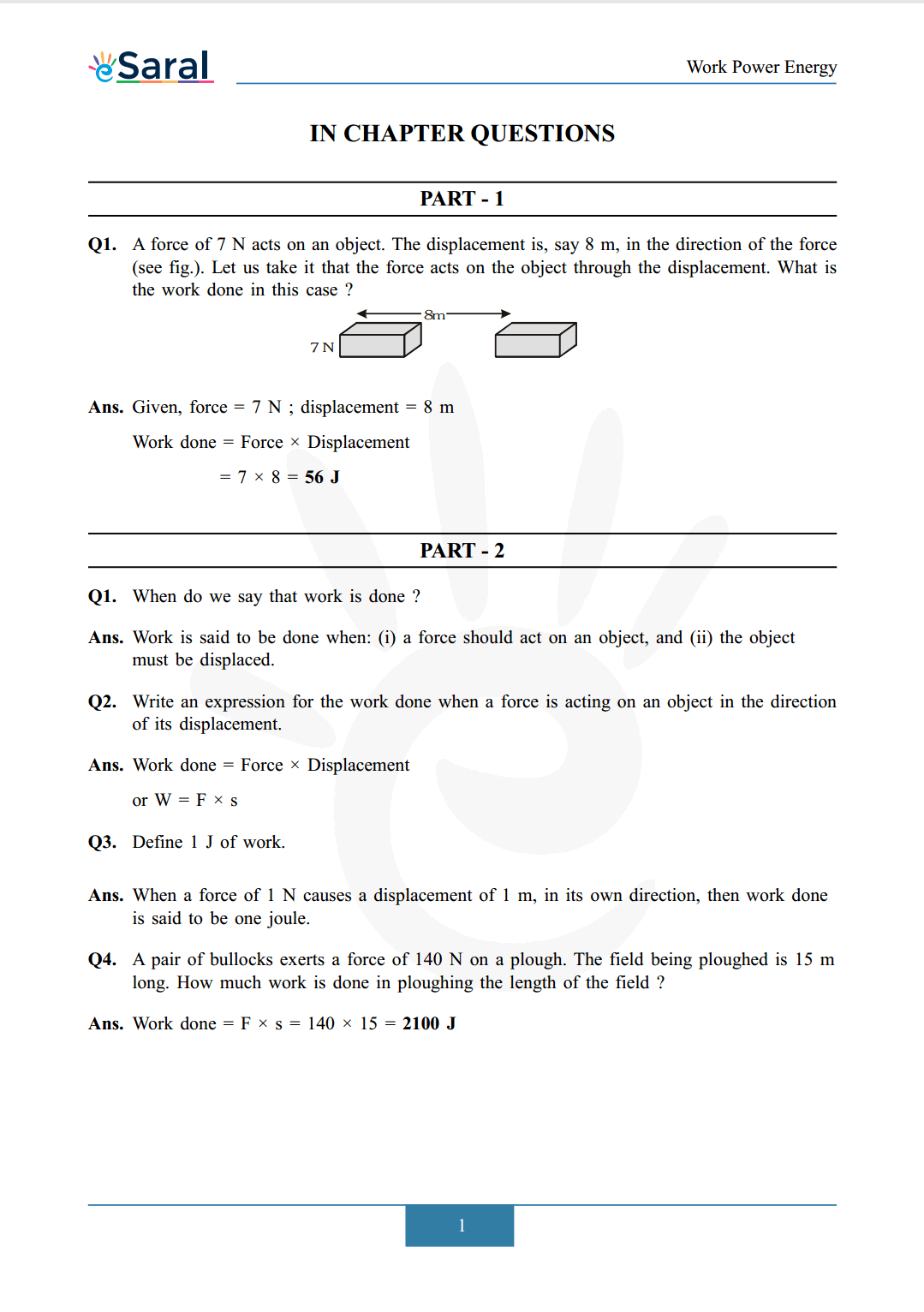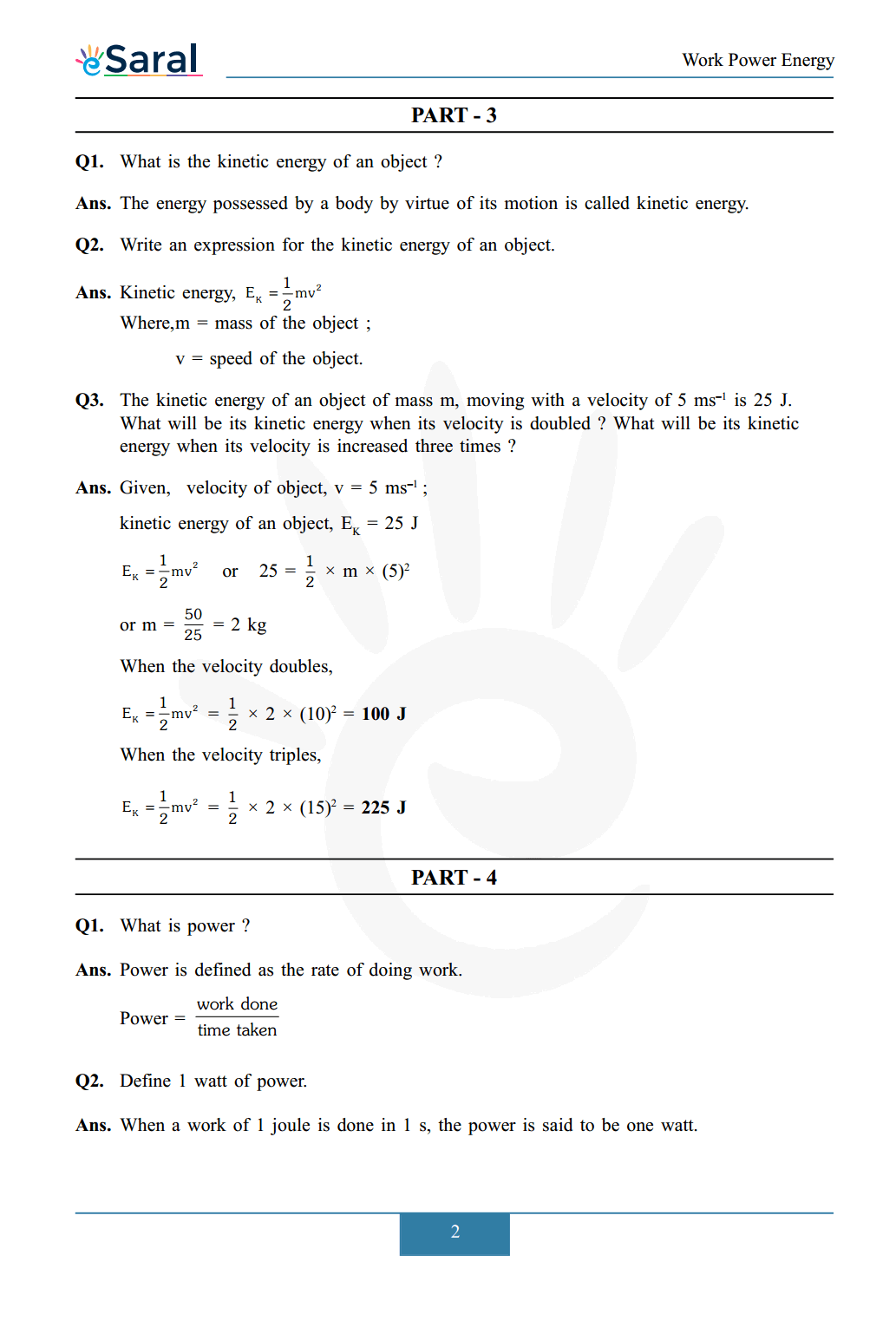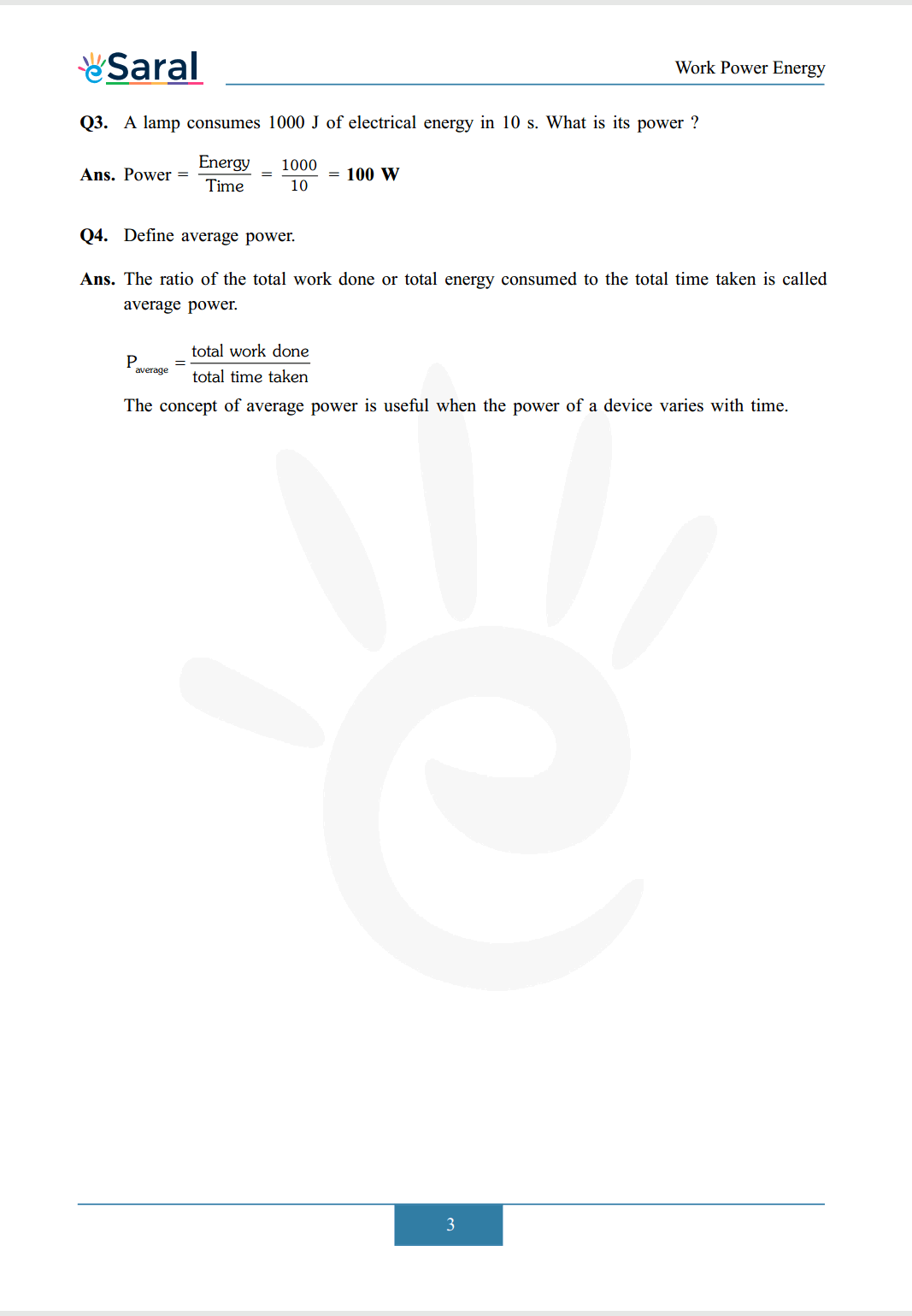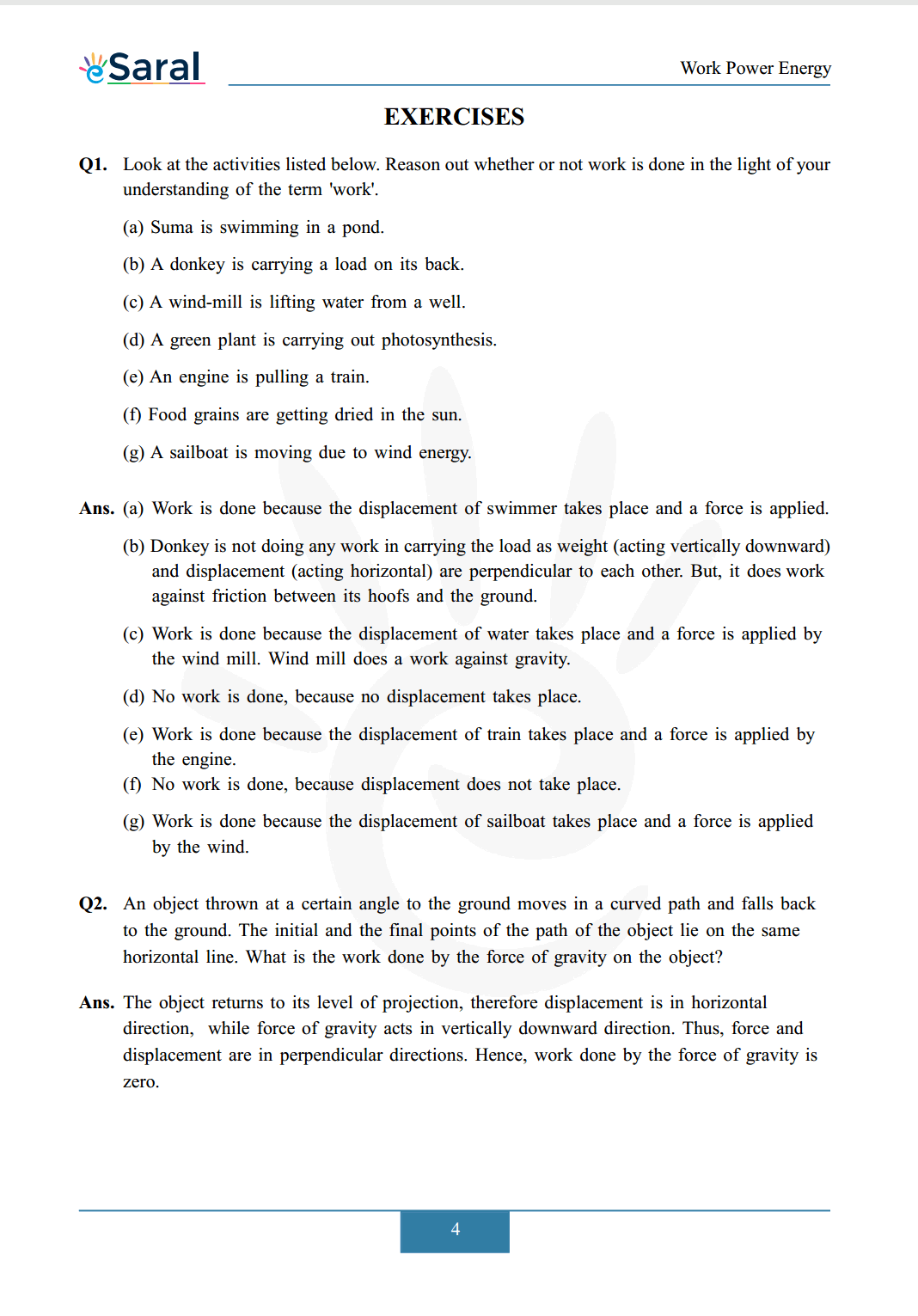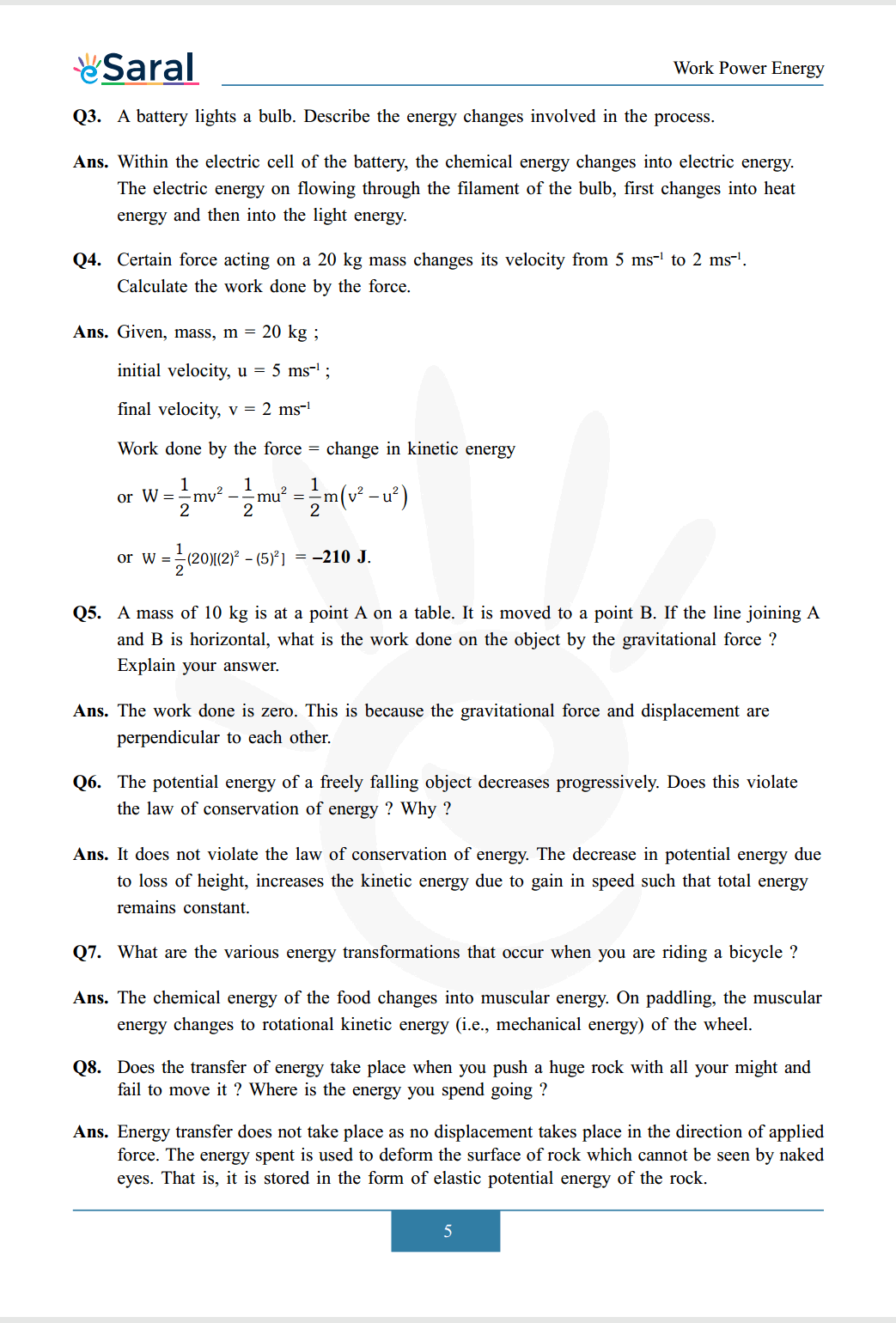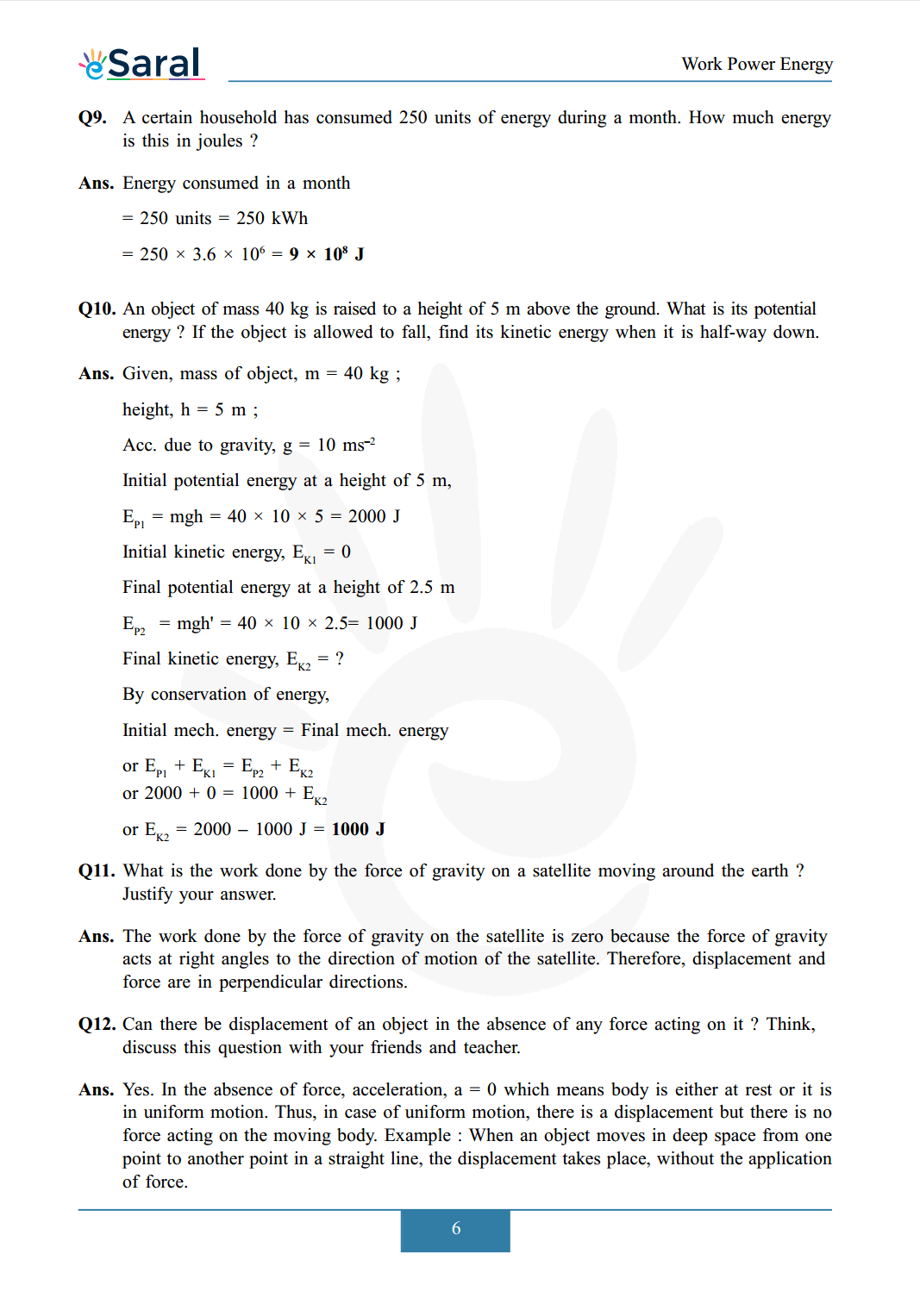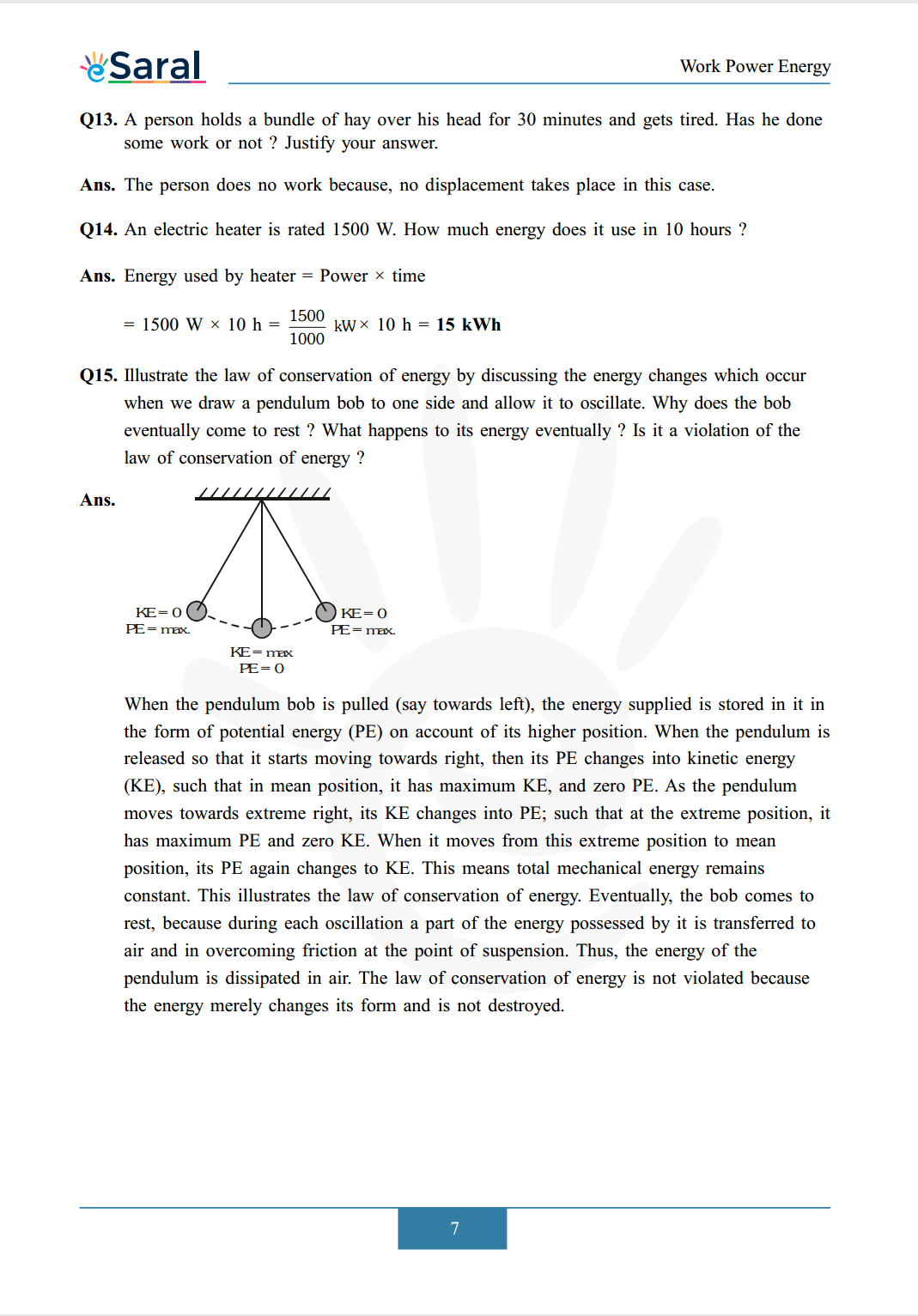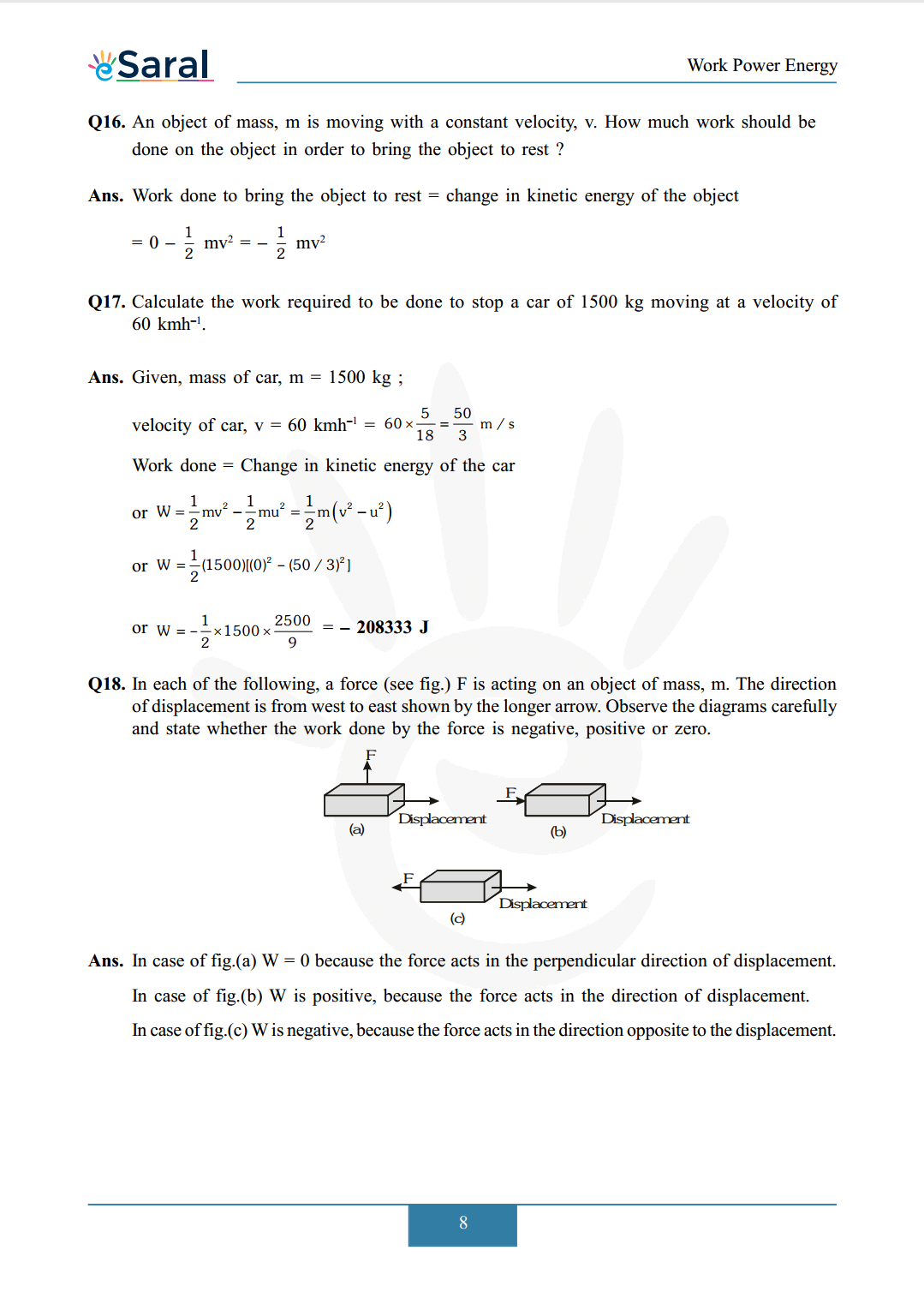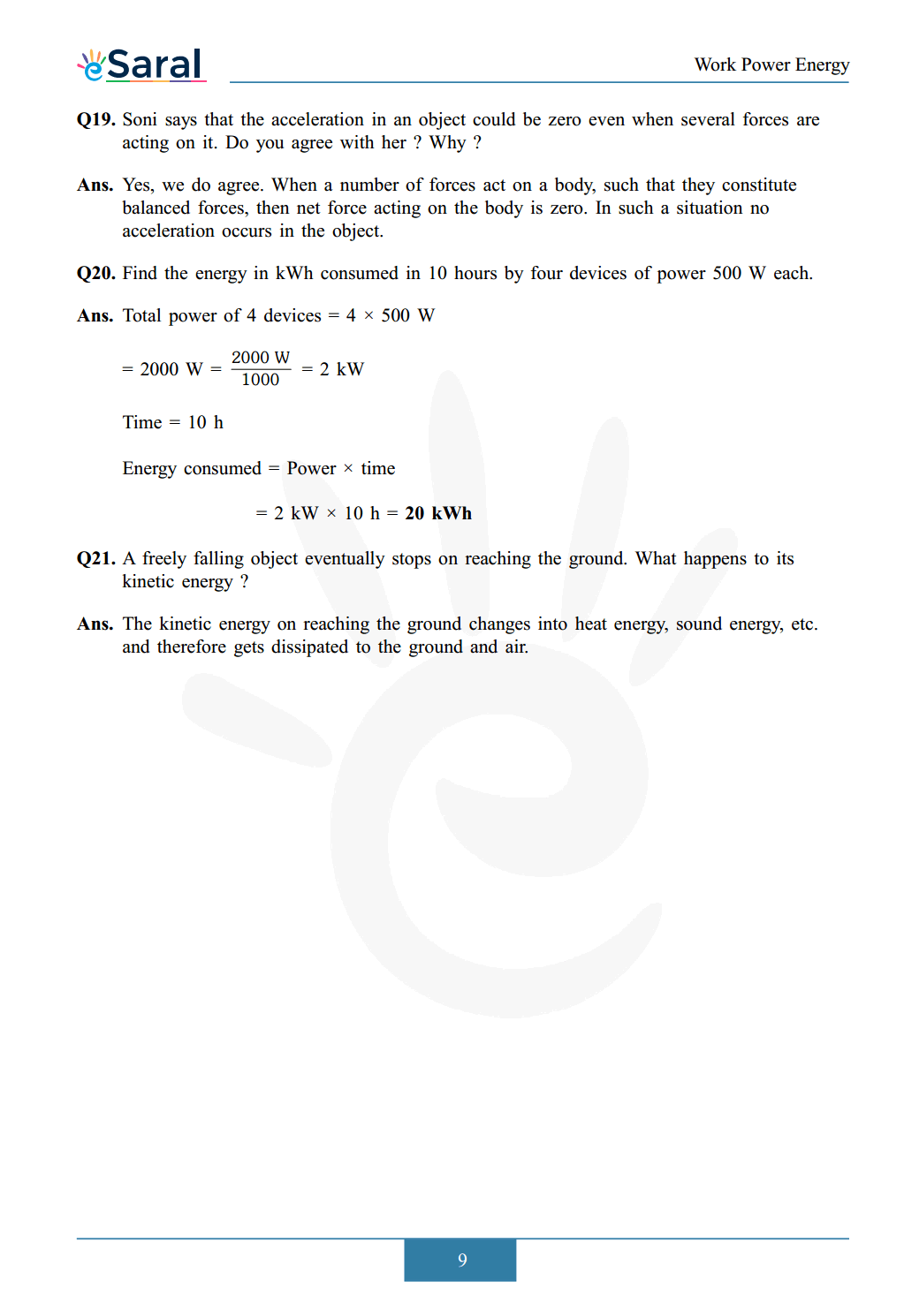Download The PDF of NCERT Solutions for Class 9 Science Chapter 11 "Work and Energy"

### Important topics of class 9 Science Chapter 11

• Work Power and Energy
• Rate of Doing Work
• Work
• Energy

#### All Questions of Chapter 11

Once you complete the chapter 11 then you can revise it by solving following questions.

Q1. A force of $7 \mathrm{~N}$ acts on an object. The displacement is, say $8 \mathrm{~m}$, in the direction of the force (see fig.). Let us take it that the force acts on the object through the displacement. What is the work done in this case ?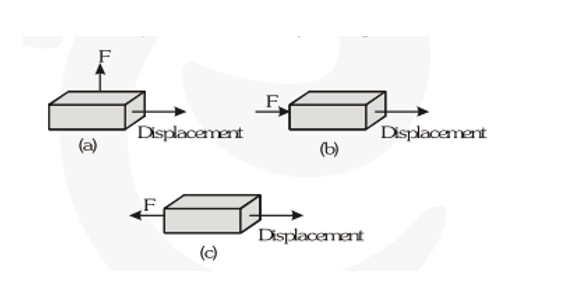##### Part 2

Q1. When do we say that work is done ?

Q2. Write an expression for the work done when a force is acting on an object in the direction of its displacement.

Q3. Define 1 J of work.

Q4. A pair of bullocks exerts a force of 140 N on a plough. The field being ploughed is 15 m long. How much work is done in ploughing the length of the field ?

##### Part 3

Q1. What is the kinetic energy of an object ?

Q2. Write an expression for the kinetic energy of an object.

Q3. The kinetic energy of an object of mass $\mathrm{m}$, moving with a velocity of $5 \mathrm{~ms}^{-1}$ is $25 \mathrm{~J}$. What will be its kinetic energy when its velocity is doubled ? What will be its kinetic energy when its velocity is increased three times ?

##### Part 4

Q1. What is power?

Q2. Define 1 watt of power.

Q3. A lamp consumes 1000 J of electrical energy in 10 s. What is its power?

Q4. Define average power.

##### EXERCISES

Q1. Look at the activities listed below. Reason out whether or not work is done in the light of your understanding of the term 'work'.

(a) Suma is swimming in a pond.
(b) A donkey is carrying a load on its back.
(c) A wind-mill is lifting water from a well.
(d) A green plant is carrying out photosynthesis.
(e) An engine is pulling a train.
(f) Food grains are getting dried in the sun.
(g) A sailboat is moving due to wind energy.

Q2. An object thrown at a certain angle to the ground moves in a curved path and falls back to the ground. The initial and the final points of the path of the object lie on the same horizontal line. What is the work done by the force of gravity on the object?

Q3. A battery lights a bulb. Describe the energy changes involved in the process.

Q4. Certain force acting on a $20 \mathrm{~kg}$ mass changes its velocity from $5 \mathrm{~ms}^{-1}$ to $2 \mathrm{~ms}^{-1}$. Calculate the work done by the force.

Q5. A mass of $10 \mathrm{~kg}$ is at a point $\mathrm{A}$ on a table. It is moved to a point $\mathrm{B}$. If the line joining $\mathrm{A}$ and $\mathrm{B}$ is horizontal, what is the work done on the object by the gravitational force ? Explain your answer.

Q6. The potential energy of a freely falling object decreases progressively. Does this violate the law of conservation of energy ? Why ?

Q7. What are the various energy transformations that occur when you are riding a bicycle ?

Q8. Does the transfer of energy take place when you push a huge rock with all your might and fail to move it ? Where is the energy you spend going ?

Q9. A certain household has consumed 250 units of energy during a month. How much energy is this in joules ?

Q10. An object of mass $40 \mathrm{~kg}$ is raised to a height of $5 \mathrm{~m}$ above the ground. What is its potential energy ? If the object is allowed to fall, find its kinetic energy when it is half-way down.

Q11. What is the work done by the force of gravity on a satellite moving around the earth ? Justify your answer.

Q12. Can there be displacement of an object in the absence of any force acting on it ? Think, discuss this question with your friends and teacher.

Q13. A person holds a bundle of hay over his head for 30 minutes and gets tired. Has he done some work or not ? Justify your answer.

Q14. An electric heater is rated 1500 W. How much energy does it use in 10 hours ?

Q15. Illustrate the law of conservation of energy by discussing the energy changes which occur when we draw a pendulum bob to one side and allow it to oscillate. Why does the bob eventually come to rest ? What happens to its energy eventually ? Is it a violation of the law of conservation of energy ?

Q16. An object of mass, $\mathrm{m}$ is moving with a constant velocity, v. How much work should be done on the object in order to bring the object to rest?

Q17. Calculate the work required to be done to stop a car of $1500 \mathrm{~kg}$ moving at a velocity of $60 \mathrm{kmh}^{-1}$

Q18. In each of the following, a force (see fig.) $\mathrm{F}$ is acting on an object of mass, $\mathrm{m}$. The direction of displacement is from west to east shown by the longer arrow. Observe the diagrams carefully and state whether the work done by the force is negative, positive or zero.Q19. Soni says that the acceleration in an object could be zero even when several forces are acting on it. Do you agree with her ? Why ?

Q20. Find the energy in kWh consumed in 10 hours by four devices of power 500 W each.

Q21. A freely falling object eventually stops on reaching the ground. What happens to its kinetic energy ?